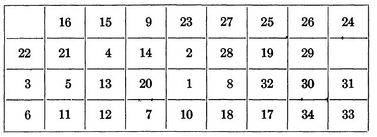# Difference between revisions of "Quadratic assignment problem"

Author: Thomas Kueny, Eric Miller, Natasha Rice, Joseph Szczerba, David Wittmann (SysEn 5800 Fall 2020)

## Theory, Methodology, and/or Algorithmic Discussions

Joe Szczerba and Tom Kueny to provide.

## Example

Consider the QAP of placing $i=3$facilities in $j=3$locations. Let the distance matrix $D_{i,j}={\begin{bmatrix}0&4.5&1.5\\4.5&0&6\\1.5&6&0\end{bmatrix}}$## Applications

Natasha Rice and David Wittmann to provide.

### The Backboard Wiring Problem

As the quadratic assignment problem is focused on minimizing the cost of traveling from one location to another, it is an ideal approach determining placement of components in many modern electronics. Leon Steinberg proposed a QAP solution optimize the layout of elements on a blackboard by minimizing the total amount of wiring required.

When defining the problem Steinberg states that we have a set of n elements

$E=\left\{E_{1},E_{2},...,E_{n}\right\}$as well as a set of r points

$P_{1},P_{2},...,P_{r}$where $r\geq n$In his paper he derives the below formula:

$min\sum _{1\leq i\leq j\leq n}^{}C_{ij}(d_{s(i)s(j))})$$C_{ij}$represents the number of wires connecting components $E_{i}$and $E_{i}$$d_{s(i)s(j))}$is the length of wire needed to connect tow components if one is placed at $P_{\alpha }$and the other is placed at $P_{\beta }$. If $P_{\alpha }$has coordinates $(x_{\alpha },y_{\alpha },z_{\alpha })$then $d_{s(i)s(j))}$can be calculated by

$d_{s(i)s(j))}=\surd ({\bigl (}x_{\alpha }-x_{\beta }{\bigr )}^{2}+{\bigl (}y_{\alpha }-y_{\beta }{\bigr )}^{2}+{\bigl (}z_{\alpha }-z_{\beta }{\bigr )}^{2})$$s(x)$represents a particular placement of a component.

In his paper Steinberg a backboard with a 9 by 4 array, allowing for 36 potential positions for the 34 components that needed to be placed on the backboard. For the calculation he selected a random initial placement of s1 and chose a random family of 25 unconnected sets.

The initial placement of components is shown below:

After the initial placement of elements it took an additional 35 iterations to get us to our final optimized backboard layout. Leading to a total of 59 iterations and a final wire length of 4,969.440.Final component position in Steinberg's Backboard optimization problem